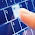## JAVA95

Let's learn java programming language with easy steps. This Java tutorial provides you complete knowledge about java technology.

# How To Convert Binary to Hexadecimal in Java

In the previous posts we have discussed some conversion programs like java program to convert decimal to binary, java program to convert binary to decimal, etc. Here we are going to discuss another conversion program which is, how to convert binary to hexadecimal in java.

Let's write a java program to convert a binary number to hexadecimal number with easy examples.

### Binary to Hexadecimal Conversion in Java

You can write a program to convert binary to hexadecimal by using 2 ways which is given below.
• By using predefined method
• By creating your own logic

Let's see first example of binary to hexadecimal conversion in java by using predefined method.

### Java Binary to Hexadecimal Example 1

This is first binary to hexadecimal example where we will take binary number from the user by using Scanner class and then convert this binary number into hexadecimal number by using predefined method.

import java.util.Scanner;
{
int number;
Scanner s;

void takeBinaryValue()
{
s = new Scanner(System.in);
System.out.println("Enter the binary number");

number = Integer.parseInt(s.nextLine(), 2);
}

void conversion()
{

String hexa = Integer.toHexString(number);
}

public static void main(String args[])
{

bh.takeBinaryValue();
bh.conversion();
}
}

Output: Enter the binary number
101010

Now we are going to take another example to convert binary to hexadecimal by creating own logic.

### Java Binary to Hexadecimal Example 2

This is another simple program to convert binary to hexadecimal in java by using self logic.

import java.util.Scanner;
{
public static void main(String args[])
{

int bnum;
int rem;
String hexanum = "";

char hex[] = {'0','1','2','3','4','5','6','7','8','9','A','B','C','D','E','F'};
Scanner sc = new Scanner(System.in);

System.out.println("Enter binary number");
bnum = sc.nextInt();

//logic for converting binary to hexadicmal number
while(bnum > 0)
{
rem = bnum%16;
hexanum = hex[rem] + hexanum;
bnum = bnum/16;
}

}
}

Output: Enter binary number
1010

Here we learned binary to hexadecimal conversion in java with simple examples.

Share:

1.Your blog is very useful and all the java examples is very well explained.

2.3.Nice article thank you.
Java Training with Placements

4.Thanks for sharing this informative blog java training in chennai